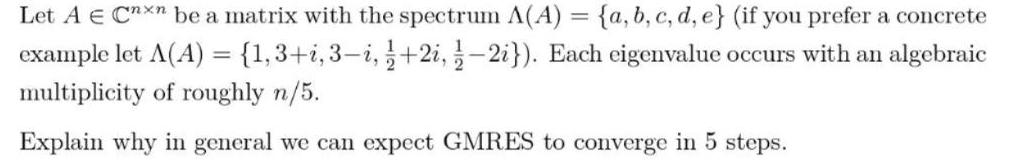Question:

# Let A € Cnxn be a matrix with the spectrum A(A) = {a, b, c,

Last updated: 7/30/2022Let A € Cnxn be a matrix with the spectrum A(A) = {a, b, c, d, e} (if you prefer a concrete example let A(A) = {1,3+i, 3-i, 1/2+2i, 1/2-2i}). Each eigenvalue occurs with an algebraic multiplicity of roughly n/5. Explain why in general we can expect GMRES to converge in 5 steps.